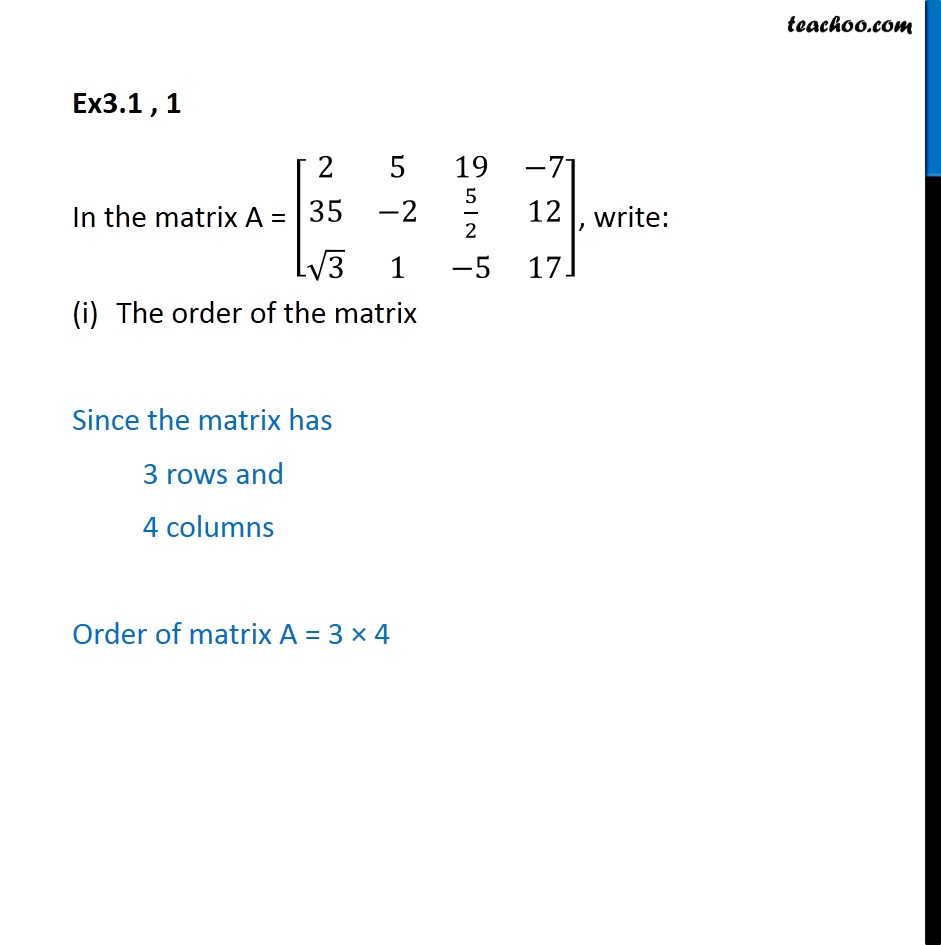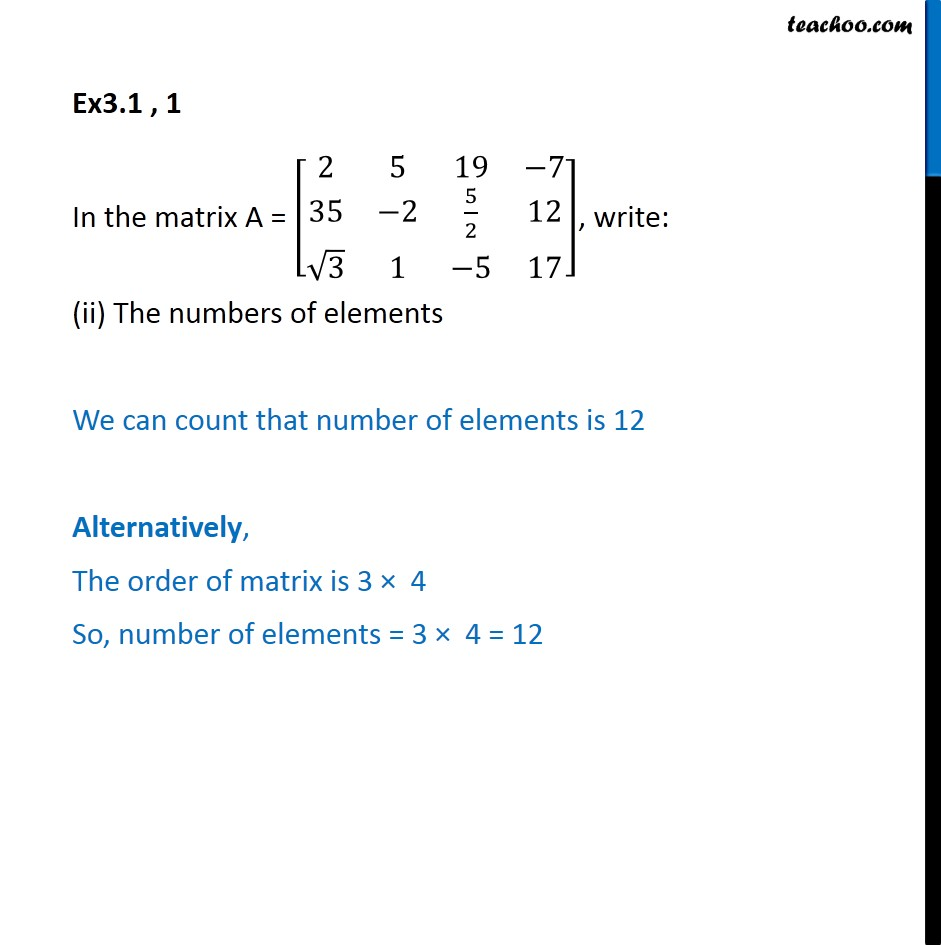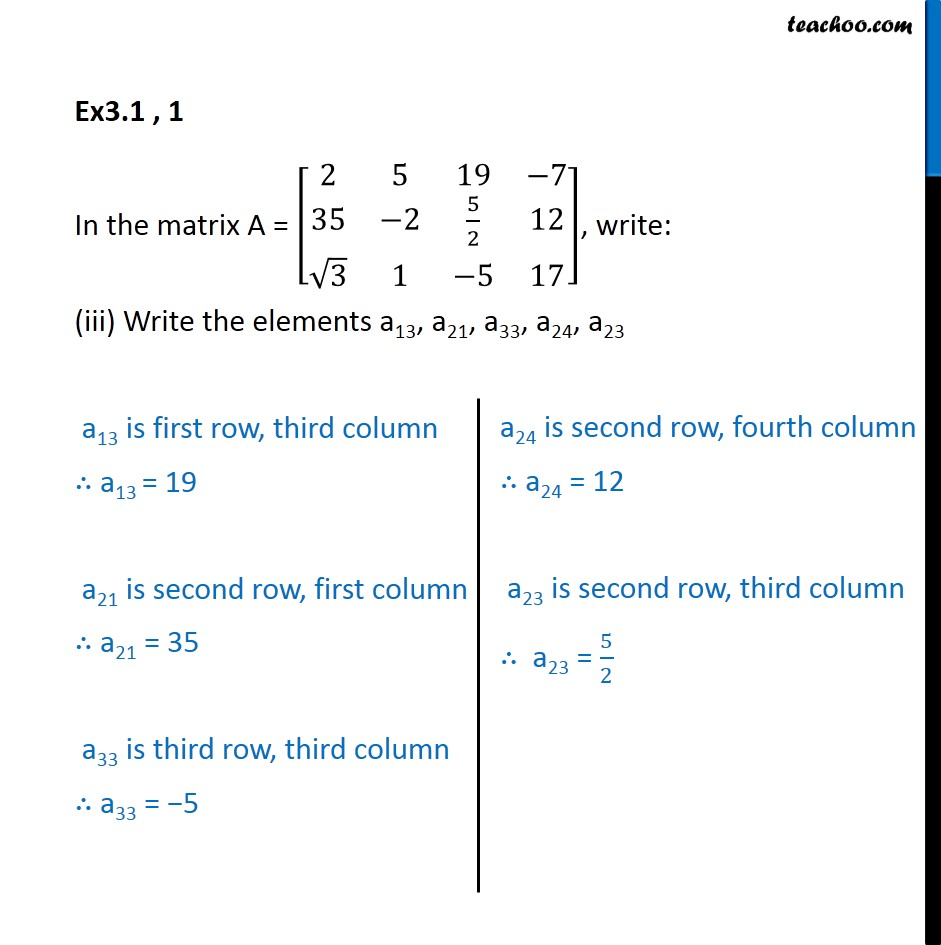1. Chapter 3 Class 12 Matrices (Term 1)
2. Serial order wise
3. Ex 3.1

Transcript

Ex3.1 , 1 In the matrix A = [■8(2&5&19&−7@35&−2&5/2&12@√3&1&−5&17)], write: The order of the matrix Since the matrix has 3 rows and 4 columns Order of matrix A = 3 × 4 Ex3.1 , 1 In the matrix A = [■8(2&5&19&−7@35&−2&5/2&12@√3&1&−5&17)], write: (ii) The numbers of elements We can count that number of elements is 12 Alternatively, The order of matrix is 3 × 4 So, number of elements = 3 × 4 = 12 Ex3.1 , 1 In the matrix A = [■8(2&5&19&−7@35&−2&5/2&12@√3&1&−5&17)], write: (iii) Write the elements a13, a21, a33, a24, a23 a13 is first row, third column ∴ a13 = 19 a21 is second row, first column ∴ a21 = 35 a33 is third row, third column ∴ a33 = −5